# Logic Dummies

When the arrow diagram was developed, there were two important problems associated with the method. The first problem is that, since an arrow served to represent both the sequence and duration of an activity, there are cases where showing sequence with a single arrow is inadequate. The diagrams below shows several examples where a single activity arrow cannot graphically express the sequence needed in an activities list.

The first example shows how Arrow Diagramming has problems representing a sequence of activities where sets of activities share some, but not all, of the prior activities. In this example, Activity C has a prior of A and Activity B has both A and B as priors.

Activity Prior Activity
A None
B None
C A
D A, B

Notice that when we try to create an Arrow Diagram with this pattern the standard notation shown in the figure below is incorrect.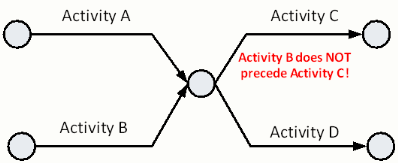The developers of the Arrow Diagram created the "logic dummy." The logic dummy, an activity with zero duration, links together activities whose sequence would otherwise not be shown. To designate the dummy activity, a dashed arrow is used. As shown on the figure below, the logic dummy is used to show the sequence between activity A and D without the problem of linking Activity B with Activity C. One way to know if a logic dummy is needed is to look at the activity list and find those activities that share some, but not the entire set of prior activities.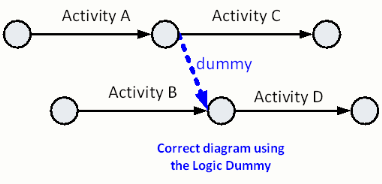Logic dummies are quite versatile. Consider the example schedule shown below. Notice that Activities D, E, and F do not all share the same set of prior activities. As a result, two logic dummy activities are needed to properly show the sequence of tasks on this project.

Activity Prior Activity
A None
B None
C None
D A, B
E B
F B, C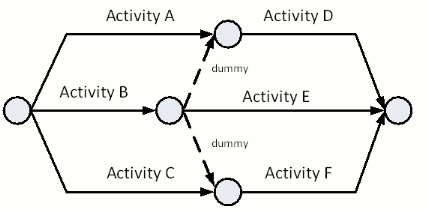Another example where logic dummies are needed is shown below. Notice that Activities C, D, and E do not share the same set of prior Activities. Since Activities C, D, and E do not share both Activities A and B as priors, logic dummies are needed to properly show the sequence using an arrow diagram.

Activity Prior Activity
A None
B None
C A
D A, B
E B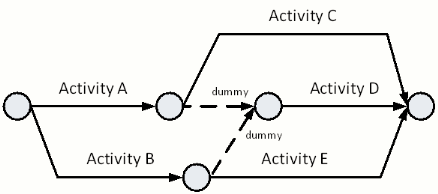While the logical dummy is a very useful tool to allow the arrow diagram to show all the required sequence contained in a set of activities, the logical dummy should only be used when necessary to show the proper sequence. The figure below gives an example of when not to use the logical dummy.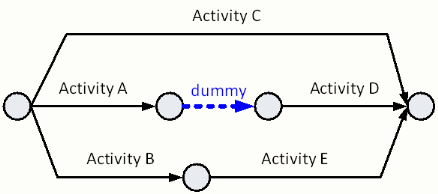In the diagram above, only prior activity to Activity D is Activity A. In this case a logic dummy is not needed between Activity A and Activity D. Logic dummies are one of the most common problem areas in the Arrow Diagramming Method. In the next section, we introduce the other area that causes problems with Arrow Diagramming, the numbering dummy.